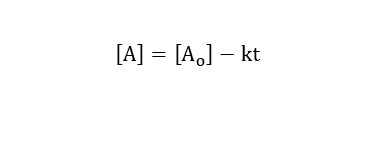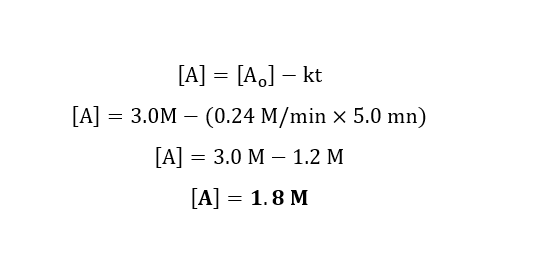# A certain reaction has k = 0.24 M/min, if 3.0 M of the reactant is initially present, how much reactants will remain after 5.0 minutes?

Question
8 views

A certain reaction has k = 0.24 M/min, if 3.0 M of the reactant is initially present, how much reactants will remain after 5.0 minutes?

check_circle

Step 1

Given:

Rate constant = 0.24 M/min

initial concentration ​[A]0 = 3.0 M

time = 5.0 minutes

We know that units of zero order reaction is M/min

Therefore, the reaction is zero order reaction.

Step 2

Zero order integrated rate law is given by:Step 3

Calculation for concentration after 5.0 minutes using zero order integrated rate law:...

### Want to see the full answer?

See Solution

#### Want to see this answer and more?

Solutions are written by subject experts who are available 24/7. Questions are typically answered within 1 hour.*

See Solution
*Response times may vary by subject and question.
Tagged in

### Chemistry